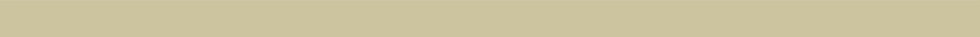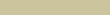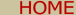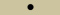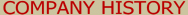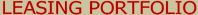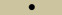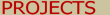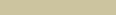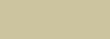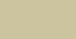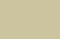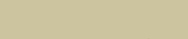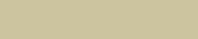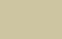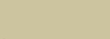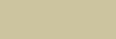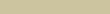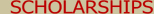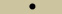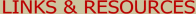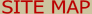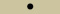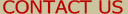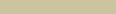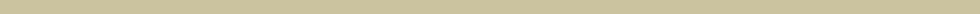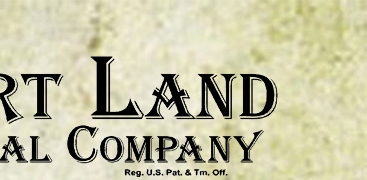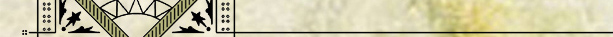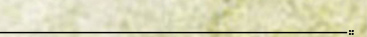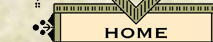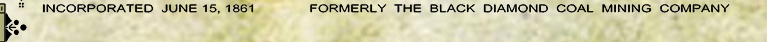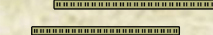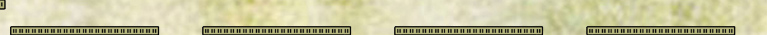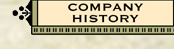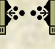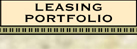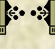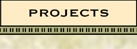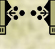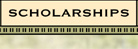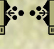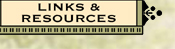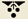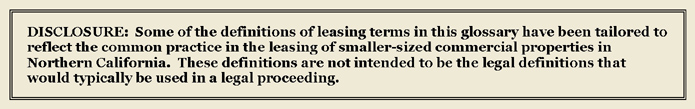# Definition of Rentable Square Feet (with examples)

“Rentable square feet” is computed by taking the square footage that is to be used exclusively by the tenant (“usable square feet”) and adding that tenant's share of the Common Area square footage.  (A tenant's share of the Common Area is the tenant's percentage of the total “usable square feet” among all tenants, multiplied by the square feet of Common Area in the building.)  The “rentable square feet” is then multiplied by the per square foot lease rate to arrive at the tenant's rent.

NOTE:  If a tenant does not have access to the entire Common Area, then the square footage of the Common Area that is available to that particular tenant, and the usable square feet of all tenants with the same type of access to the common area, should be used in the calculations.  If all tenants have access to some Common Area, and less than all tenants have access to a different Common Area, then separate calculations need to be performed on each distinct portion of the Common Area.  (See examples below.)

### Example Calculations of Rentable Square Feet

SIMPLE EXAMPLE:  Assume that a building has 4,000 square feet of usable square feet among all units, and 1,000 square feet of total Common Area.  Also assume that Unit A in the building has 2,000 square feet of usable square feet.  The per square foot lease rate is \$1.65 per month.  The calculations would be as follows:
Calculate Unit A's percent of usable square feet: 2,000 ÷ 4,000 = 50%.

Calculate Unit A's per square foot share of common area: 50% x 1,000 = 500 square feet.

Calculate Unit A's rentable square feet: 2,000 (usable) + 500 (share of total common area) = 2,500.

Calculate Unit A's monthly rent: \$1.65 x 2,500 = \$4,125 per month.

SLIGHTLY MORE COMPLEX EXAMPLE:  Assume that a building has 4,000 square feet of usable square feet among all units, and 1,000 square feet of total Common Area.  Unit A and Unit B in the building can only use 400 square feet of the Common Area.  Unit A has 2,000 square feet of usable square feet, while Unit B has 750 square feet of usable square feet.  The per square foot lease rate is \$1.65 per month.  The calculations would be as follows:

Calculate Unit A's percent of usable square feet: 2,000 ÷ 2,750 = 72.73%.

Calculate Unit A's per square foot share of available common area: 72.73% x 400 = 291 square feet.

Calculate Unit A's rentable square feet: 2,000 (usable) + 291 (share of available common area) = 2,291.

Calculate Unit A's monthly rent: \$1.65 x 2,291 = \$3,780 per month.

COMPLEX EXAMPLE:  Assume that a building has 4,000 square feet of usable square feet among all units.  There are two distinct areas of Common Area, a restroom and hallway of 400 square feet available only to Unit A and Unit B, and a trash room and hallway of 600 square feet available to all units in the building.  Unit A has 2,000 square feet of usable square feet, while Unit B has 750 square feet of usable square feet.  The per square foot lease rate is \$1.65 per month.  The calculations would be as follows:

Calculate Unit A's percent of usable square feet as to “restroom” common area: 2,000 ÷ 2,750 = 72.73%.

Calculate Unit A's per square foot share of “restroom” common area: 72.73% x 400 = 291 square feet.

Calculate Unit A's percent of usable square feet as to “trash room” common area: 2,000 ÷ 4,000 = 50%.

Calculate Unit A's per square foot share of “trash room” common area: 50% x 600 = 300 square feet.

Calculate Unit A's rentable square feet: 2,000 (usable) + 291 (share of “restroom” common area) + 300 (share of “trash room” common area) = 2,591.

Calculate Unit A's monthly rent: \$1.65 x 2,591 = \$4,275 per month.